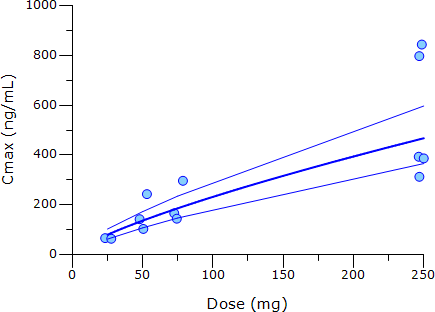## Setup in Phoenix/WinNonlin [Study As­sess­ment]

Hi Angus,

❝ It is a very well known drug and many MR formulations are on the market. (You have experience with this drug).

If we are talking about the same goody: Watch out for polymorphism… Sometimes you have poor metabolizers in the study where enzymes get saturated at higher doses. In one subject I once got a slope of 1.54 for AUC and 2.16 for Cmax over a only twofold dose range! For the other subjects it got 1.05 and 1.02 with a very narrow CI.

❝ There are only two does levels to plot. The relationship is as follows:

❝ LnPK=B0+B1*Ln(dose) where LnPK pertains to Cmax or AUC.

❝ So we have a regression line going through the points: we evaluate the slope (B1), intercept

OK, so far.

❝ and the confidence intervals about the slope to evaluate dose proportionality.

This is beyond me. df = np where n is the number of data points and p the number of parameters. How can you calculate a CI with df = 0?

❝ Brian Smith in Pharm Research year 2000 has extended the approach from the original UK working party.

Yep. Smith et al. use a mixed-effects model, where subjects are a random effect. Thus we increase n. Now a CI is possible even for p = 2.

❝ It seems that you can calculate intrasubject and intersubject variance e.g. for AUC and partial AUC from this approach.

Correct.

❝ I do not follow how to do it. I use the usual intrasubject and intersubject values from Phoenix WinNonlin 6.4 and I am happy with that.

If you are happy with that, what is your question?If you want to reproduce Smith’s results in Phoenix/WinNonlin: Start with a worksheet (columns subject, dose, Cmax, AUC, whatsoever). log-transform: dose, Cmax, … and weight=1/logCmax, … Send to LME. Map Subject as Classification, logCmax as Rgeressor, and logCmax as Dependent.
Model Specification: logCmax
Fixed Effects Confidence Level: 90%
Variance Structure / Random 1: Subject
With Smith’s Cmax-data of Table 1 I got for the slope:
0.7617 (90% CI: 0.6696, 0.8539), slightly different from the reported 0.7615 (0.679, 0.844). Why? Duno.See also chapter 18.3 in Chow/Liu. Without explanation they recommend a 95% CI but a 90% CI in elaborating Smith’s approach. In general I prefer a weighted model (hence the transformation above). Fits much better.
                 SSQ      AIC   Var(Subject)  Var(Res) w = 1          0.07389   9.247     0.1120     0.01456  w = 1/logCmax  0.01548  -8.917     0.1108     0.003076

PS: Can you ask “the other worker” why he/she calculated the 98% CI?

Dif-tor heh smusma 🖖🏼 Довге життя Україна!Helmut SchützThe quality of responses received is directly proportional to the quality of the question asked. 🚮
Science QuotesIng. Helmut Schütz